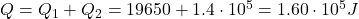Question

How much energy must be transferred away from .5kg of 10 degrees Celsius milk in order for it to turn into ice cream? Assume the heat of fusion for milk is 2.8×10^5 J/kg. The specific heat for milk is 3930 J/kgC.

1.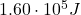Explanation:

First of all, we have to calculate the amount of energy that must be transferred away from the milk in order to bring it to the freezing temperature, zero degrees. This is given by: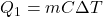where:

m = 0.5 kg is the mass of milk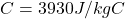is the specific heat of milk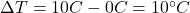is the change in temperature

Substituting,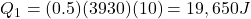Then, after the milk has reached its freezing temperature, the amount of energy that must be removed in order to turn it into ice cream (convert it from liquid to solid) is given by: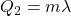where

m = 0.5 kg is the mass of milk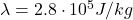is the specific heat of fusion

Substituting,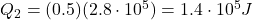Therefore, the total heat needed is